Vibroengineering PROCEDIA

Published: 22 October 2022

# Experimental study on creep wetting of silty clay

Dahe Qi1
Jianxin Yang2
Yuehan Liu3
1, 3Chongqing Metropolitan College of Science and Technology, ChongQing, China
2Hunan Lixian Housing and Urban Rural Development Bureau, Lixian, China
Corresponding Author:
Dahe Qi
Views 8

#### Abstract

To study the wetting of a silty clay subgrade in an expressway, many laboratory tests were carried out. The test results show that the creep deformation of silty clay under water immersion cannot be ignored, but it is different from the deformation caused by wetting. The creep properties of soil mass are affected by many factors. Under different stress conditions, the soil mass will show different properties. In order to study the creep characteristics of silty clay in Chongqing under water wetting and different stress levels, a series of triaxial water wetting creep tests were carried out. The Singh-Mitchell creep equation was introduced to establish the creep model of silty clay drainage in Chongqing, and the parameters of the model were discussed.

#### Highlights

• The silty clay along the Jialing River in Chongqing has obvious creep characteristics.
• Calculated the parameters of the Singh-Mitchell model to describe the creep wetting properties of silty clay.

## 1. Introduction

Many scholars has studied the subgrade soil, such as coastal soft soil subgrade [3-5], soft clay and structural clay  in different areas. The above researches have promoted the study of creep characteristics of different soil mass, but most of them consider the creep test of soil mass under a single stress state . In fact, in view of the fact that the creep phenomenon of soil is mostly viscoelastic and plastic, and the stress state has a significant impact on the creep characteristics of soil, it is of great significance to study the creep characteristics of silty clay at different stress levels.

This paper takes the silty clay widely distributed in Chongqing area as the research object. Through triaxial drained shear creep tests under different stress levels, it focuses on demonstrating the creep characteristics of silty clay after wetting in water, and discusses its corresponding suitable model, so as to understand its deformation mechanism in depth.

## 2. Silty clay sample properties and test method

### 2.1. Silty clay sample

The silty clay soil samples used in the test were taken from the undisturbed silty clay samples within 3.5-6 m below the subgrade of a highway along the Jialing River in Chongqing, that were quaternary Holocene alluvium silty clay (q4al), basic physical properties see Table 1.

Table 1Basic physical property of soil samples

 Density Moisture content Dry density Proportion g/cm3 % g/cm3 1.82 20.10 1.52 2.734 Liquid limit Plastic limit Saturated moisture Void ratio % % % 38.74 19.16 29.36 0.803

### 2.2. Test method

The test in this paper was carried out at 22±2℃, and the creep test was carried out by means of graded loading. The confining pressures are: 100 kPa, 200 kPa and 300 kPa, the stress level , respectively 0.2, 0.35, 0.5, 0.75. The silty clay soil sample was fixed with hoops and splints, placed on filter paper and permeable stone, add axial pressure and confining pressure. when the total axial stress $D$ reaches 0.2 of the total stress at the time of drainage shear failure, that is, when the stress level $D$ is 0.2, stop pressurizing and maintain the pressure unchanged, the sample starts to saturate from bottom to top, meanwhile the wetting creep test data was recorded.

According to the author’s previous test results , The axial failure stress of silty clay under various confining pressures is 240 kPa, 430 kPa and 620 kPa respectively. The test process is as follows:

(1) Install the sample and apply confining pressure to consolidate the sample;

(2) Take the readings measured after consolidation as the initial readings of the triaxial creep test, and then apply the vertical axial stress $q$;

(3) After the incremental load is applied, observe the change process of axial direction deformation with time, and record the creep deformation data;

(4) When the deformation is stable, apply the next level of load.

## 3. Analysis of creep test results

### 3.1. Creep stress-strain curve

The creep stress-strain curve of the creep test is shown in Fig. 1, the curve is processed by Chen’s method . Under the same confining pressure, the silty clay creep deformation amplitude at low stress level is small, the stress-strain curve is almost a straight line. At higher stress level, the strain gradually increases with time, and the creep deformation also increases.

At the same stress level $D$, the creep deformation and rate under high confining pressure are larger than those under lower. When the stress level $D=$ 0.75, the creep rate far exceeds other stress levels.

Fig. 1Creep stress-strain curves under different confining pressures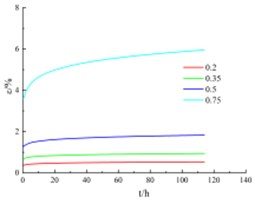a) Confining pressure = 100 kPa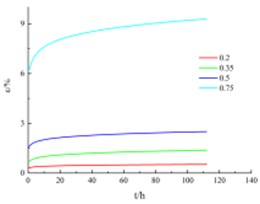b) Confining pressure = 200 kPa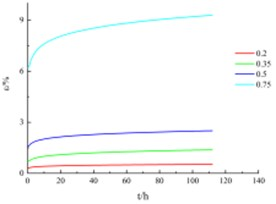b) Confining pressure = 300 kPa

### 3.2. Creep isochronous stress-strain curve

The shape of stress-strain isochronous curves at different times is shown in Fig. 2, which indicates that silty clay has obvious creep characteristics. When time $t=$ 0, the s stress-strain isochronous curve is approximately a straight line, so it can be considered that the initial deformation is elastic deformation.

When time 0, with the extension of creep time, the isochronous stress-strain curve gradually deviates from the straight line and bends toward to the strain axis, and the longer the creep time, the higher the degree of deviation of the isochronous curve from the straight line, and the more obvious the bending toward to the direction of the strain axis.

The test results show that, from the beginning of loading, the stress-strain isochrone curve shows obvious nonlinear characteristics, and its nonlinear degree is related to creep time and stress level. The higher the stress level $D$ is, the more obvious the axial bending toward the strain axis, the longer the creep time, the higher the nonlinear extent.

Fig. 2Stress-strain isochronous curves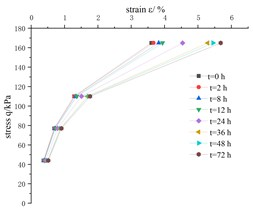a) Confining pressure = 100 kPa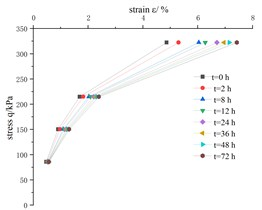b) Confining pressure = 200 kPa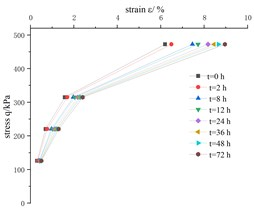c) Confining pressure = 300 kPa

## 4. Applicable model of creep stress-strain curve

At present, there are three ways to describe the creep characteristics of rock and soil: theoretical model based on model theory, empirical model based on test results and micro model derived from micro rheology. The former two approaches are most widely used.

A. Singh and J.K. Mittechell  proposed the classical Singh-Mitchell creep model on the basis of summarizing the single-stage constant stress loading, drained and undrained triaxial compression test data, and proposed that the stress-strain relationship can be expressed by an exponential relationship and the strain time relationship can be expressed by a power function, which is a three parameters model used to describe the deviator stress level within 20 %-80 %, and can well reflect the creep characteristics of soil. The equation of Singh Mitchell creep model is as follows:

1
$\epsilon ={\epsilon }_{0}+B{e}^{\beta \stackrel{-}{D}}{\left(\frac{t}{{t}_{1}}\right)}^{1-m}.$

When ${\epsilon }_{0}=0$, the Eq. (1) can be expressed as:

2
$\epsilon =B{e}^{\beta \stackrel{-}{D}}{\left(\frac{t}{{t}_{1}}\right)}^{\lambda },$

where, $B=A{t}_{1}/1-m$, $\beta =\alpha$, $\lambda =1-m$, $m$ – absolute value of the slope of the straight line in the relation curve of $\mathrm{l}\mathrm{o}\mathrm{g}\stackrel{˙}{\epsilon }~\mathrm{l}\mathrm{o}\mathrm{g}t$.

This is the mathematical expression of the Singh-Mitchell model. When expressed by strain, there are three parameters $B$, $\beta$, $\lambda$, the model parameters can be determined according to the test results. Creep test can be controlled by drainage conditions, so the Singh-Mitchell model can describe both drainage conditions and undrained conditions .

When $t={t}_{1}$, the Eq. (2) can be expressed as exponential stress-strain relation:

3
$\epsilon =B{e}^{\beta \stackrel{-}{D}}.$

Take the logarithm on both sides:

4
$\mathrm{l}\mathrm{n}\epsilon =\beta \stackrel{-}{D}+\mathrm{l}\mathrm{n}B.$

Fig. 3The logε~logt curve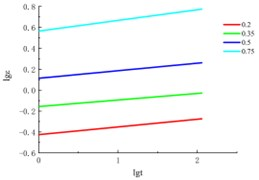a) Confining pressure = 100 kPa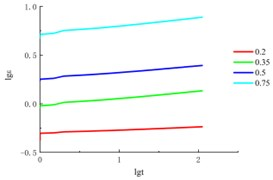b) Confining pressure = 200 kPa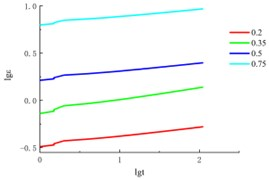b) Confining pressure = 300 kPa

Based on the above formulas and creep test results, $B$ can be directly obtained from the relationship curve of soil samples at different times, $\lambda$ is the slope of the model parameter straight line is related to the deviator stress $D$, which can be taken as the average value when the difference is not large, calculation results see in Table 2. Collating the creep data, draw the curve, as shown in the Fig. 3.

Table 2λ calculation result

 Confining pressure (kPa) $\stackrel{-}{\mathrm{\lambda }}$ 100 0.072575 200 0.07185 300 0.105425

Fig. 4The lnε~D- curve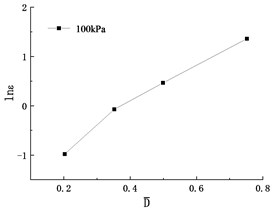a) Confining pressure = 100 kPa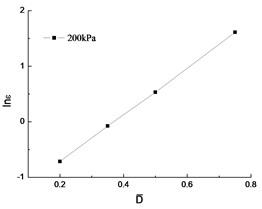b) Confining pressure = 200 kPa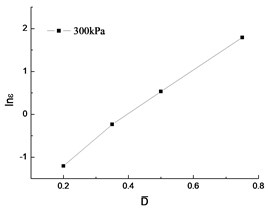b) Confining pressure = 300 kPa

Parameter $B$, $\beta$ can be directly obtained from the relationship curve of $\mathrm{l}\mathrm{n}\epsilon ~\stackrel{-}{D}$ in Fig. 4, the linear relationship is relatively good. Table 3 shows the calculation results.

Table 3Parameter B, β

 Confining pressure (kPa) $B$ $\beta$ ${R}^{2}$ 100 4.1502 0.1631 0.9815 200 4.2119 0.21081 0.9998 300 5.3856 0.1105 0.9966

The Singh Mitchell model equation of the creep stress-strain curve of silty clay is:

5
6
7

This model can well describe the creep stress-strain curve of silty clay. The larger the stress level, the worse the data consistency. If the stress level exceeds 0.8, there will be a large deviation, the Singh-Mitchell model is not suitable for describing the creep curve.

## 5. Conclusions

In this paper, the triaxial creep wetting test was successfully carried out, and the creep deformation characteristics were revealed.

1) The silty clay of road subgrade along the Jialing River in Chongqing has obvious creep characteristics.

2) Use the graded loading method, obtained sufficient creep test data, which provides a basis for testing the accuracy of the creep model.

3) Introduced the Singh-Mitchell model to describe the creep stress-strain curve, calculated the parameters $\left(\lambda ,\beta ,B\right)$ of silty clay.

In the actual project, the structure of soil mass must be fully obtained. Necessary supporting structures must be added to control the creep deformation. Next study will focus the creep deformation of silty clay under cyclic loading for a longer time.

#### Acknowledgements

Our research was supported by the Science and Technology Research Program of Chongqing Municipal Education Commission (Grant No. KJQN202202503). More in-depth research will be conducted in the future.